# Transistor Biasing & Stabilization Notes | Study Analog Electronics - Electrical Engineering (EE)

## Electrical Engineering (EE): Transistor Biasing & Stabilization Notes | Study Analog Electronics - Electrical Engineering (EE)

The document Transistor Biasing & Stabilization Notes | Study Analog Electronics - Electrical Engineering (EE) is a part of the Electrical Engineering (EE) Course Analog Electronics.
All you need of Electrical Engineering (EE) at this link: Electrical Engineering (EE)

NEED FOR TRANSISTOR BIASING:

If the o/p signal must be a faithful reproduction of the i/p signal, then transistor must be operated in active region. That means an operating point has to be established in this region . To establish an operating point (proper values of collector current IC and collector to emitter voltage VCE) appropriate supply voltages and resistances must be suitably chosen in the circuit. This process of selecting proper supply voltages and resistance for obtaining desired operating point or Q point is called as biasing and the circuit used for transistor biasing is called as biasing circuit.

There are four conditions to be met by a transistor so that it acts as a faithful amplifier:

1. Emitter base junction must be forward biased (VBE=0.7Vfor Si, 0.2V for Ge) and collector base junction must be reverse biased for all levels of i/p signal.
2. Vce voltage  should not fall below VCE (sat) (0.3V for Si, 0.1V for Ge) for any part of the i/p signal. For VCE less than VCE (sat) the collector base junction is not probably reverse biased.
3. The value of the signal Ic when no signal is applied should be at least equal to the max. collector current due to signal alone.
4. Max. rating of the transistor Ic(max), VCE (max) and PD(max) should not be exceeded at any value of i/p signal.

Consider fig1. If operating point is selected at A, A represents a condition when no bias is applied to the transistor i.e, IC=0, VCE =0. It does not satisfy the above stated conditions necessary for faithful amplification.

Point C is too close to PD(max) curve of the transistor. Therefore  the o/p voltage swing in the positive direction is limited.

Point B is located in the middle of active region. It will allow both positive and negative  half cycles in the o/p signal. It also provides linear gain and larger possible o/p voltages and currents.

Hence operating point for a transistor amplifier is selected to be in the middle of active region.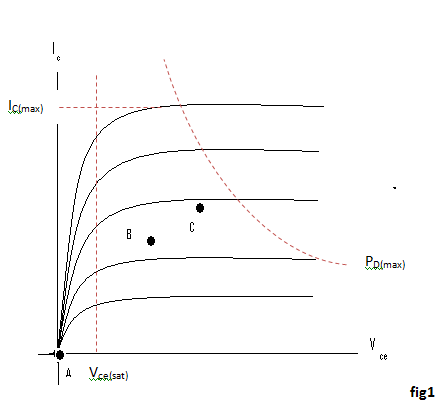Referring to the biasing circuit of fig 4.2a, the values of VCC and RC are fixed and IC and VCE are dependent on RB.

Applying Kirchhoff’s voltage law to the collector circuit in fig. 4.2a, we get

VCC= ICR+ Vce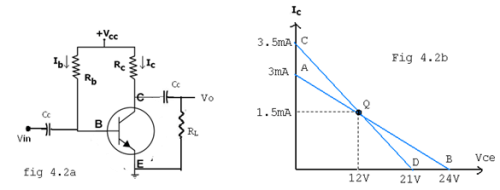The straight line represented by AB in fig4.2b is called the dc load line. The coordinates of the end point A are obtained by substituting VCE =0 in the above equation. Then

ICC = VCC/RC  Therefore The coordinates of A are  VCE =0 and ICC = VCC/RC.

The coordinates of B are obtained by substituting IC=0 in the above equation. Then Vce = Vcc. Therefore the coordinates of B are VCE =Vcc and IC=0. Thus the dc load line AB can be drawn if the values of RC and VCC are known.

As shown in the fig4.2b, the optimum POINT IS LOCATED AT THE MID POINT OF THE MIDWAY BETWEEN A AND B. In order to get faithful amplification, the Q point must be well within the active region of the transistor.

Even though the Q point is fixed properly, it is very important to ensure that the operating point remains stable where it is originally fixed. If the Q point shifts nearer to either A or B, the output voltage and current get clipped, thereby distorting the o/p signal.

In practice, the Q-point tends to shift its position due to any or all of the following three main factors.

1. Reverse saturation current, Ico, which doubles for every 10oC raise in temperature
2. Base emitter Voltage ,VBE, which decreases by 2.5 mV per oC
3. Transistor current gain, hFE or β which increases with temperature.

If base current IB is kept constant since IB is approximately equal to Vcc/RB. If the transistor is replaced by another one of the same type, one cannot ensure that the new transistor will have identical parameters as that of the first one. Parameters such as β vary over a range. This results in the variation of collector current IC for a given IB. Hence , in the o/p characteristics, the spacing between the curves might increase or decrease which leads to the shifting of the Q-point to a location which might be completely unsatisfactory.

After drawing the dc load line, the operating point Q is properly located at the center of the dc load line. This operating point is chosen under zero input signal condition of the circuit. Hence the ac load line should also pass through the operating point Q. The effective ac load resistance Rac, is a parallel combination of RC and RL i.e. Rac = R|| RC. So the slope of the ac load line CQD will be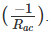. To draw the ac load line, two end points, i.e. VCE(max) and IC(max)  when the signal is applied are required.

VCE(max)=VCEQ+ICQRac, which locates point D on the Vce axis.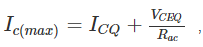which locates the point C  on the IC axis.

By joining points C and D, ac load line CD is constructed. As RC > Rac, The dc load line is less steep than ac load line.

STABILITY FACTOR (S):

The rise of temperature results in increase in the value of transistor gain β and the leakage current ICO. So, IC also increases which results in a shift in operating point. Therefore, The biasing network should be provided with thermal stability. Maintenance of the operating point is specified by S, which indicates the degree of change in operating point due to change in temperature.

The extent to which IC is stabilized with varying temperature is measured by a stability factor S.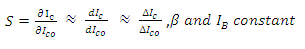For CE configuration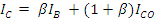Differentiate the above equation w.r.t IC , We get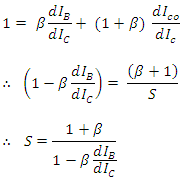S should be small to have better thermal stability.

Stability factor S’ and S’’:

S’ is defined as the rate of change of   IC with VBE, keeping IC and VBE constant.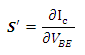S’’ is defined as the rate of change of   IC with β, keeping ICO and VBE constant.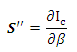The document Transistor Biasing & Stabilization Notes | Study Analog Electronics - Electrical Engineering (EE) is a part of the Electrical Engineering (EE) Course Analog Electronics.
All you need of Electrical Engineering (EE) at this link: Electrical Engineering (EE)Use Code STAYHOME200 and get INR 200 additional OFF

### Up next## Analog Electronics

11 videos|57 docs|97 tests

### Up nextTrack your progress, build streaks, highlight & save important lessons and more!

,

,

,

,

,

,

,

,

,

,

,

,

,

,

,

,

,

,

,

,

,

;